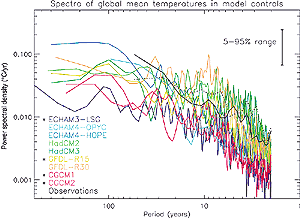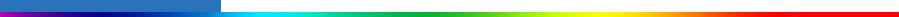Working Group I: The Scientific BasisGet Javascript Other reports in this collection

Continued from previous page

Estimates of the variability of global mean surface temperatureFigure 12.2: Coloured lines: power spectra of global mean temperatures in the unforced control integrations that are used to provide estimates of internal climate variability in Figure 12.12. All series were linearly detrended prior to analysis, and spectra computed using a standard Tukey window with the window width (maximum lag used in the estimate) set to one-fifth of the series length, giving each spectral estimate the same uncertainty range, as shown (see, e.g., Priestley, 1981). The first 300 years were omitted from ECHAM3-LSG, CGCM1 and CGCM2 models as potentially trend-contaminated. Solid black line: spectrum of observed global mean temperatures (Jones et al., 2001) over the period 1861 to 1998 after removing a best-fit linear trend. This estimate is unreliable on inter-decadal time-scales because of the likely impact of external forcing on the observed series and the negative bias introduced by the detrending. Dotted black line: spectrum of observed global mean temperatures after removing an independent estimate of the externally forced response provided by the ensemble mean of a coupled model simulation (Stott et al., 2000b, and Figure 12.7c). This estimate will be contaminated by uncertainty in the model-simulated forced response, together with observation noise and sampling error. However, unlike the detrending procedure, all of these introduce a positive (upward) bias in the resulting estimate of the observed spectrum. The dotted line therefore provides a conservative (high) estimate of observed internal variability at all frequencies. Asterisks indicate models whose variability is significantly less than observed variability on 10 to 60 year time-scales after removing either a best-fit linear trend or an independent estimate of the forced response from the observed series. Significance is based on an F-test on the ratio observed/model mean power over this frequency interval and quoted at the 5% level. Power spectral density (PSD) is defined such that unit-variance uncorrelated noise would have an expected PSD of unity (see Allen et al., 2000a, for details). Note that different normalisation conventions can lead to different values, which appear as a constant offset up or down on the logarithmic vertical scale used here. Differences between the spectra shown here and the corresponding figure in Stouffer et al. (2000) shown in Chapter 8, Figure 8.18 are due to the use here of a longer (1861 to 2000) observational record, as opposed to 1881 to 1991 in Figure 8.18. That figure also shows 2.5 to 97.5% uncertainty ranges, while for consistency with other figures in this chapter, the 5 to 95% range is displayed here.

Stouffer et al. (2000) assess variability simulated in three 1,000-year control simulations (see Figure 12.1). The models are found to simulate reasonably well the spatial distribution of variability and the spatial correlation between regional and global mean variability, although there is more disagreement between models at long time-scales (>50 years) than at short time-scales. None of the long model simulations produces a secular trend which is comparable to that observed. Chapter 8, Section 8.6.2. assesses model-simulated variability in detail. Here we assess the aspects that are particularly relevant to climate change detection. The power spectrum of global mean temperatures simulated by the most recent coupled climate models (shown in Figure 12.2) compares reasonably well with that of detrended observations (solid black line) on interannual to decadal time-scales. However, uncertainty of the spectral estimates is large and some models are clearly underestimating variability (indicated by the asterisks). Detailed comparison on inter-decadal time-scales is difficult because observations are likely to contain a response to external forcings that will not be entirely removed by a simple linear trend. At the same time, the detrending procedure itself introduces a negative bias in the observed low-frequency spectrum.

Both of these problems can be avoided by removing an independent estimate of the externally forced response from the observations before computing the power spectrum. This independent estimate is provided by the ensemble mean of a coupled model simulation of the response to the combination of natural and anthropogenic forcing (see Figure 12.7c). The resulting spectrum of observed variability (dotted line in Figure 12.2) will not be subject to a negative bias because the observed data have not been used in estimating the forced response. It will, however, be inflated by uncertainty in the model-simulated forced response and by noise due to observation error and due to incomplete coverage (particularly the bias towards relatively noisy Northern Hemisphere land temperatures in the early part of the observed series). This estimate of the observed spectrum is therefore likely to overestimate power at all frequencies. Even so, the more variable models display similar variance on the decadal to inter-decadal time-scales important for detection and attribution.

Estimates of spatial patterns of variability
Several studies have used common empirical orthogonal function (EOF) analysis to compare the spatial modes of climate variability between different models. Stouffer et al. (2000) analysed the variability of 5-year means of surface temperature in 500-year or longer simulations of the three models most commonly used to estimate internal variability in formal detection studies. The distribution of the variance between the EOFs was similar between the models and the observations. HadCM2 tended to overestimate the variability in the main modes, whereas GFDL and ECHAM3 underestimated the variability of the first mode. The standard deviations of the dominant modes of variability in the three models differ from observations by less than a factor of two, and one model (HadCM2) has similar or more variability than the observations in all leading modes. In general, one would expect to obtain conservative detection and attribution results when natural variability is estimated with such a model. One should also expect control simulations to be less variable than observations because they do not contain externally forced variability. Hegerl et al. (2000) used common EOFS to compare 50-year June-July-August (JJA) trends of surface temperature in ECHAM3 and HadCM2. Standard deviation differences between models were marginally larger on the 50-year time-scale (less than a factor of 2.5). Comparison with direct observations cannot be made on this time-scale because the instrumental record is too short.

Variability of the free atmosphere
Gillett et al. (2000a) compared model-simulated variability in the free atmosphere with that of detrended radiosonde data. They found general agreement except in the stratosphere, where present climate models tend to underestimate variability on all time-scales and, in particular, do not reproduce modes of variability such as the quasi-biennial oscillation (QBO). On decadal time-scales, the model simulated less variability than observed in some aspects of the vertical patterns important for the detection of anthropogenic climate change. The discrepancy is partially resolved by the inclusion of anthropogenic (greenhouse gas, sulphate and stratospheric ozone) forcing in the model. However, the authors also find evidence that solar forcing plays a significant role on decadal time-scales, indicating that this should be taken into account in future detection studies based on changes in the free atmosphere (see also discussion in Chapter 6 and Section 12.2.3.1 below).

Comparison of model and palaeoclimatic estimates of variability
Comparisons between the variability in palaeo-reconstructions and climate model data have shown mixed results to date. Barnett et al. (1996) compared the spatial structure of climate variability of coupled climate models and proxy time-series for (mostly summer) decadal temperature (Jones et al., 1998). They found that the model-simulated amplitude of the dominant proxy mode of variation is substantially less than that estimated from the proxy data. However, choosing the EOFs of the palaeo-data as the basis for comparison will maximise the variance in the palaeo-data and not the models, and so bias the model amplitudes downwards. The neglect of naturally forced climate variability in the models might also be responsible for part of the discrepancy noted in Barnett et al. (1996) (see also Jones et al., 1998). The limitations of the temperature reconstructions (see Chapter 2, Figure 2.21), including for example the issue of how to relate site-specific palaeo-data to large-scale variations, may also contribute to this discrepancy. Collins et al. (2000) compared the standard deviation of large-scale Northern Hemisphere averages in a model control simulation and in tree-ring-based proxy data for the last 600 years on decadal time-scales. They found a factor of less than two difference between model and data if the tree-ring data are calibrated such that low-frequency variability is better retained than in standard methods (Briffa et al., 2000). It is likely that at least part of this discrepancy can be resolved if natural forcings are included in the model simulation. Crowley (2000) found that 41 to 69% of the variance in decadally smoothed Northern Hemisphere mean surface temperature reconstructions could be externally forced (using data from Mann et al. (1998) and Crowley and Lowery (2000)). The residual variability in the reconstructions, after subtracting estimates of volcanic and solar-forced signals, showed no significant difference in variability on decadal and multi-decadal time-scales from three long coupled model control simulations. In summary, while there is substantial uncertainty in comparisons between long-term palaeo-records of surface temperature and model estimates of multi-decadal variability, there is no clear evidence of a serious discrepancy.

Summary
These findings emphasise that there is still considerable uncertainty in the magnitude of internal climate variability. Various approaches are used in detection and attribution studies to account for this uncertainty. Some studies use data from a number of coupled climate model control simulations (Santer et al., 1995; Hegerl et al., 1996, 1997, North and Stevens, 1998) and choose the most conservative result. In other studies, the estimate of internal variance is inflated to assess the sensitivity of detection and attribution results to the level of internal variance (Santer et al., 1996a, Tett et al., 1999; Stott et al., 2001). Some authors also augment model-derived estimates of natural variability with estimates from observations (Hegerl et al., 1996). A method for checking the consistency between the residual variability in the observations after removal of externally forced signals (see equation A12.1.1, Appendix 12.1) and the natural internal variability estimated from control simulations is also available (e.g., Allen and Tett, 1999). Results indicate that, on the scales considered, there is no evidence for a serious inconsistency between the variability in models used for optimal fingerprint studies and observations (Allen and Tett, 1999; Tett et al., 1999; Hegerl et al., 2000, 2001; Stott et al., 2001). The use of this test and the use of internal variability from the models with the greatest variability increases confidence in conclusions derived from optimal detection studies.